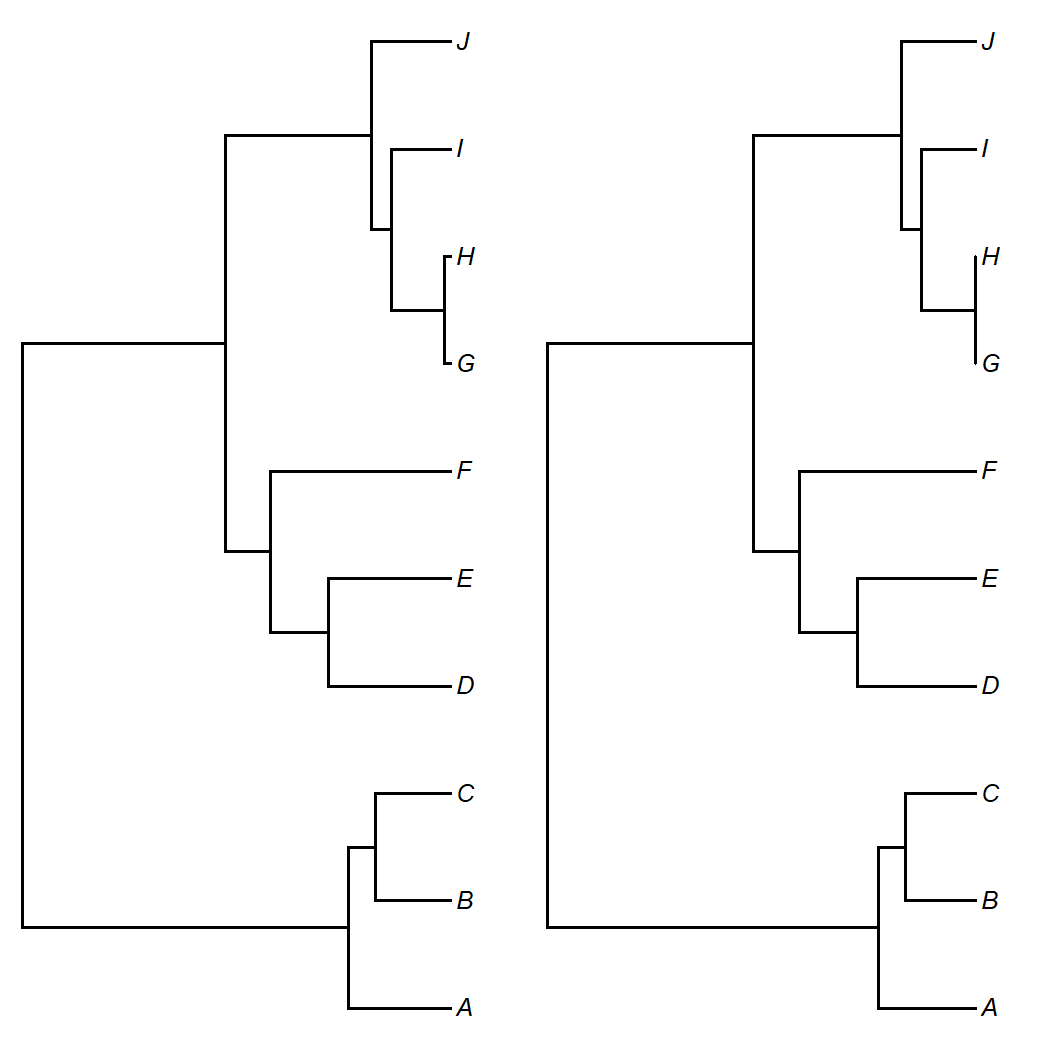## Saturday, June 4, 2022

### A pernicious phylogenetic artifact that can result in wildly different phylogenetic signal measures from K vs. λ

A not uncommon discovery by phytools users interested in the measurement of phylogenetic signal is that λ and Blomberg et al.'s K can often assume very different values for the same data and tree.

I usually point out that this is mostly due to the fact that K and λ measure phylogenetic signal in really different ways: K as a (normalized) variance ratio, comparing the variance among clades to that within clades; and λ as a tree transformation.

Recently, though, it occurred to me that K can be subject to a particularly pernicious type of data artifact.

First, consider the two phylogenetic trees shown below.

``````library(phytools)
par(mfrow=c(1,2))
plotTree(tree1,ftype="i")
plotTree(tree2,ftype="i")
``````Almost identical, right?

Now consider the following trait vector, `x`, simulated on the leftward of the two trees from the previous figure.

``````x
``````
``````##          A          B          C          D          E          F          G
##  0.5827564 -0.9664150 -0.3607281 -3.2014217 -1.8584430  0.4136624 -3.1747904
##          H          I          J
## -3.4582838 -3.1313907 -2.6239279
``````

One would think that phylogenetic signal measured using the tree on the left vs. the tree on the right would be almost identical, given their very close resemblance. Unfortunately, one would be wrong.

``````phylosig(tree1,x,method="K",test=TRUE)
``````
``````##
## Phylogenetic signal K : 1.16472
## P-value (based on 1000 randomizations) : 0.007
``````
``````phylosig(tree2,x,method="K",test=TRUE)
``````
``````##
## Phylogenetic signal K : 0.0291587
## P-value (based on 1000 randomizations) : 0.117
``````

We see that for the first tree, measured K is around 1.16: very close to our Brownian motion expectation and highly significant. K for the tree on the right, however, is nearly zero and non-significant.

What happened? Well, what I did to get the tree on the right from that on the left was simply shorten all the terminal edges of the tree by just slightly less than the tip lengths of H and G. This has the effect of massively inflating the measured variance of this clade, since it has to be normalized by the expected variance separating the two taxa. This goes from some measurable amount to nearly zero between the two trees!

Now let's try with Pagel's λ. Since λ is a tree transformation that (for λ<1) lengthens the terminal edge lengths of the tree, we would expect it to be less sensitive to this issue.

``````phylosig(tree1,x,method="lambda",test=TRUE)
``````
``````##
## Phylogenetic signal lambda : 1.00251
## logL(lambda) : -14.8423
## LR(lambda=0) : 6.75817
## P-value (based on LR test) : 0.00933192
``````
``````phylosig(tree2,x,method="lambda",test=TRUE)
``````
``````##
## Phylogenetic signal lambda : 0.98808
## logL(lambda) : -14.8423
## LR(lambda=0) : 6.75817
## P-value (based on LR test) : 0.00933192
``````

In fact, that's exactly what we find!

The reason that I refer to this as an “artifact” is because it will typically result when we underestimate the phylogenetic distance between two terminal taxa in our tree. (This actually tends to happen alot with phylogenies inferred from molecular data.) It can also occur if we don't take error in the estimation of species means into account as this can result in a larger perceived difference between closely related species than their true dissimilarity.

What do you think? Have you seen this in your own data?

For the record, the tree and data for this example were simulated as follows:

``````set.seed(30)
tree1<-pbtree(n=10,tip.label=LETTERS[1:10])
x<-fastBM(tree1)
slice<-floor(min(tree1\$edge.length)*10000)/10000
tree2<-treeSlice(tree1,max(nodeHeights(tree1))-slice,
orientation="rootwards")
``````